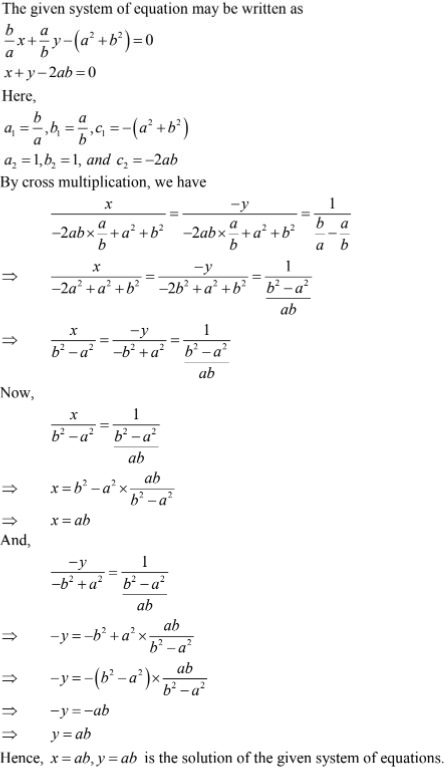# RD Sharma Solutions Chapter 3 Pair of Linear Equation in Two Variables Exercise 3.4 Class 10 Maths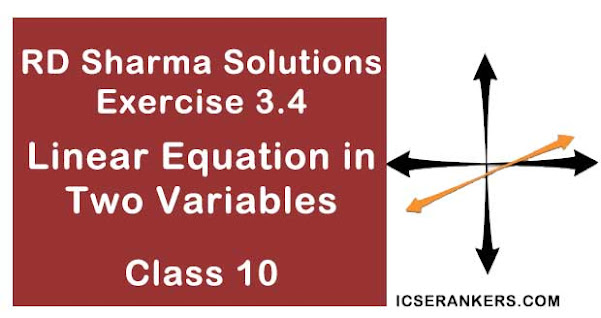Chapter Name RD Sharma Chapter 3 Pair of Linear Equation in Two Variables Book Name RD Sharma Mathematics for Class 10 Other Exercises Exercise 3.1Exercise 3.2Exercise 3.3Exercise 3.5Exercise 3.6 Related Study NCERT Solutions for Class 10 Maths

### Exercise 3.4 Solutions

Solve each of the following systems of equations by the method of cross - multiplication:

1. x + 2y + 1 = 0
2x - 3y - 12 = 0

Solution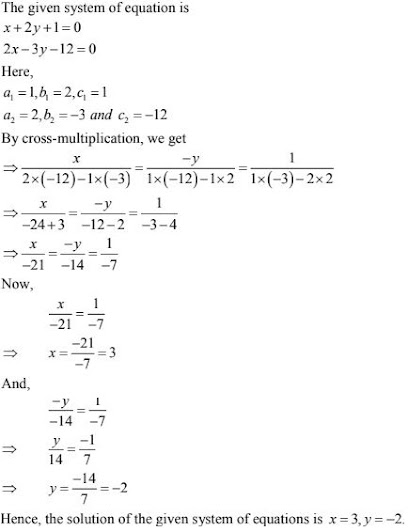2. 3x + 2y + 25 = 0
2x + y + 10 = 0

Solution

The given system of equation is
3x + 2y + 25 = 0
2x + y + 10 = 0
Here,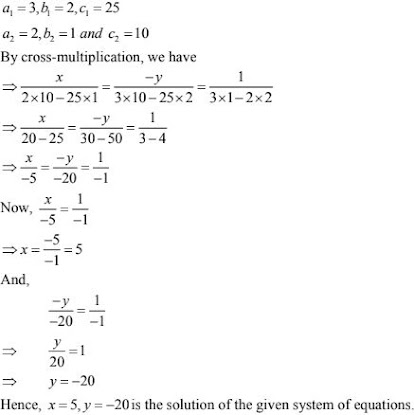3. 2x + y - 35 = 0
3x + 4y - 65 = 0

Solution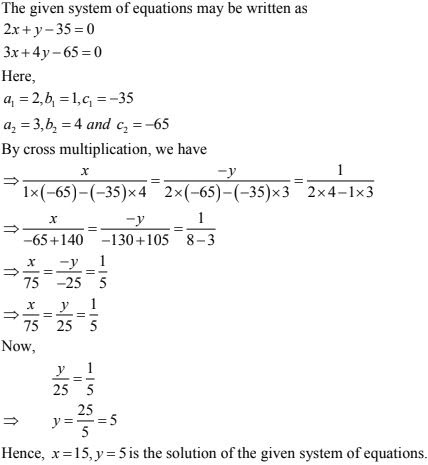4. 2x - y - 6 = 0
x - y - 2 = 0

Solution

The given system of equations may be written as
2x - y - 6 = 0
x - y - 2 = 0
Here,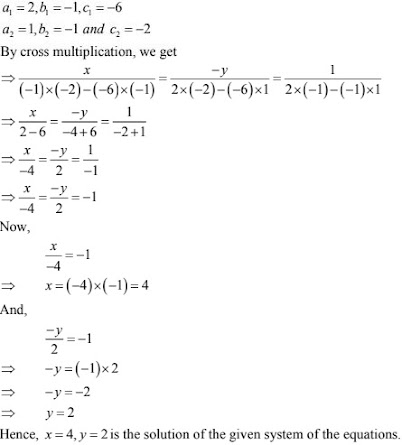5. (x+y)/xy = 2
(x-y)/xy = 6

Solution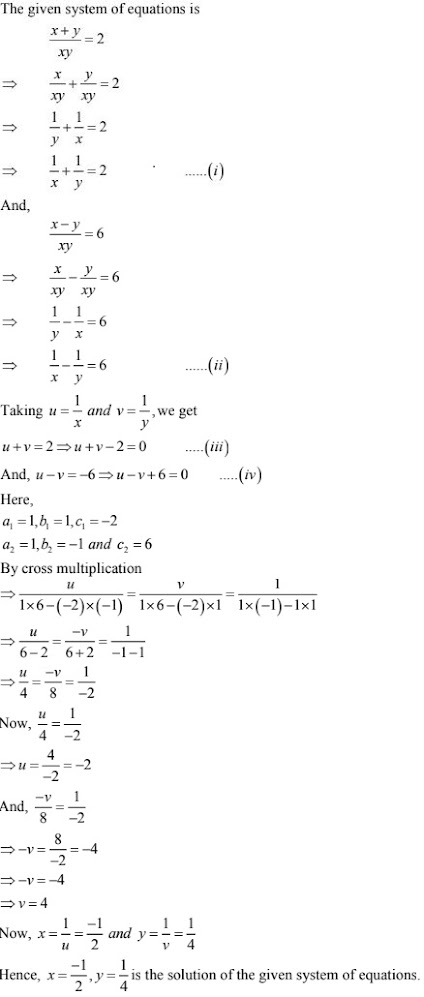6. ax + by = a-b
bx - ay = a + b

Solution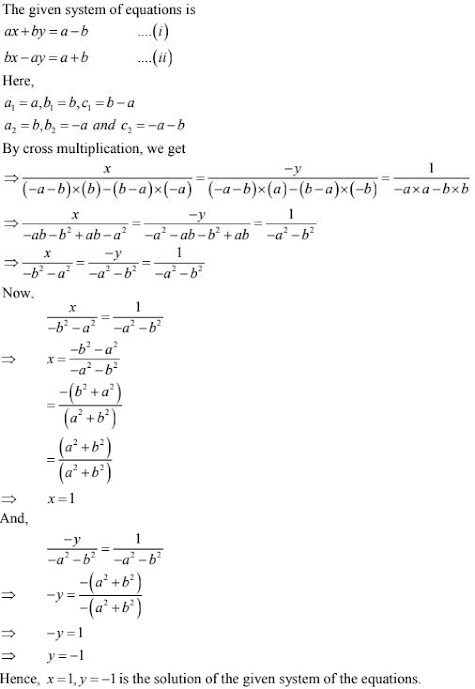7. x + ay - b = 0
ax - by -c = 0

Solution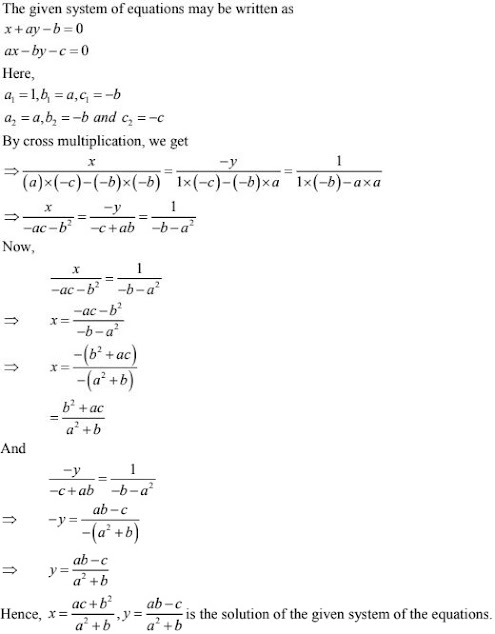8. ax + by = a2 .
bx + ay = b2

Solution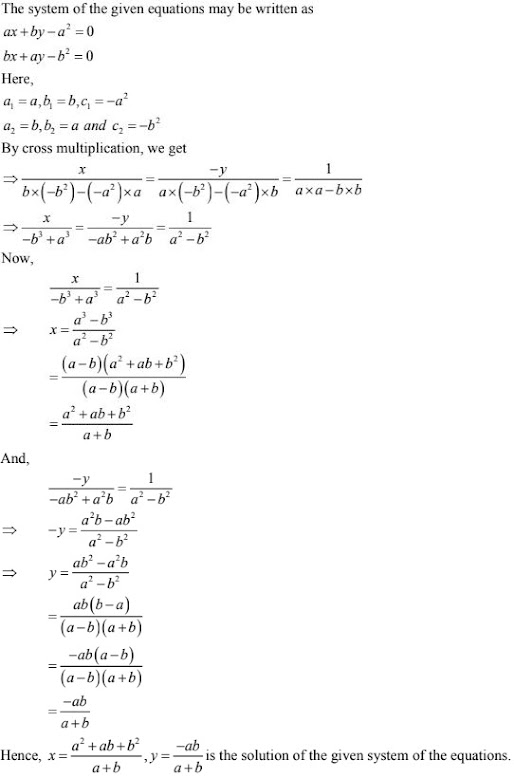9. x/a + y/b = 2
ax - by = a2 - b2

Solution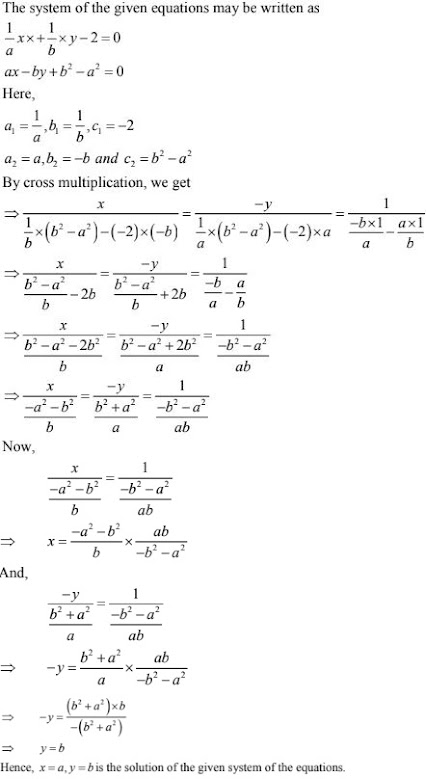10. x/a + y/b = a + b

Solution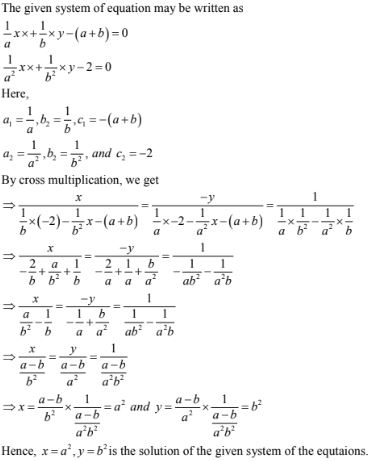11. x/a = y/b
ax + by = a2 + b2

Solution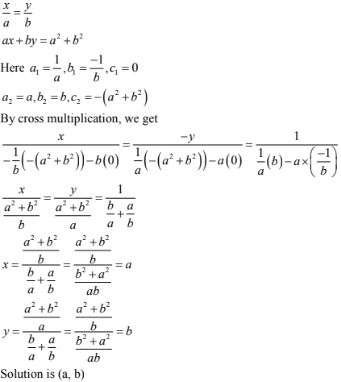12. 5/(x+y) - 2/(x -y) = -1
15/(x + y) + 7/(x - y) = 10, where x ≠ 0 and  y ≠ 0

Solution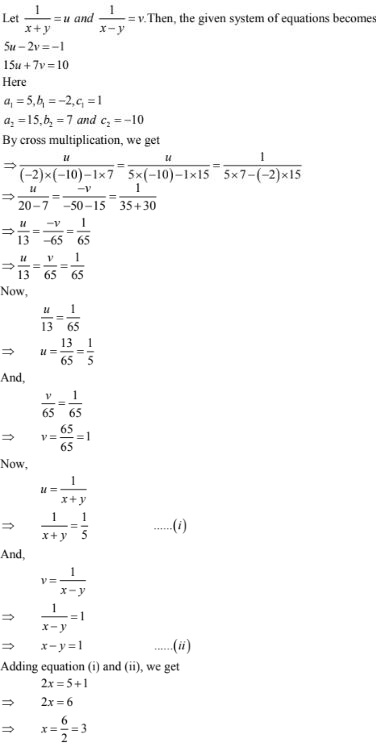13. 2/x + 3/y = 13
5/x - 4/y = -2 , where x ≠ 0 and y ≠ 0

Solution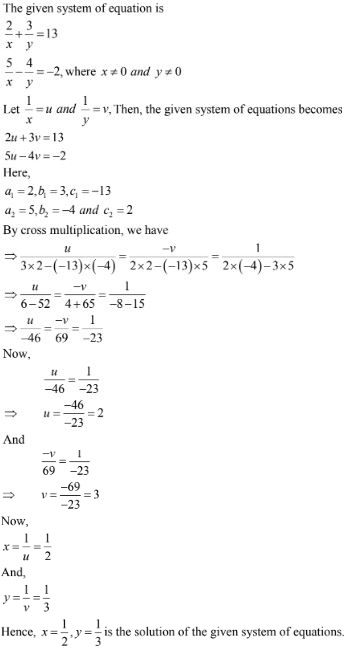14. ax + by = (a + b)/2
3x + 5y = 4

Solution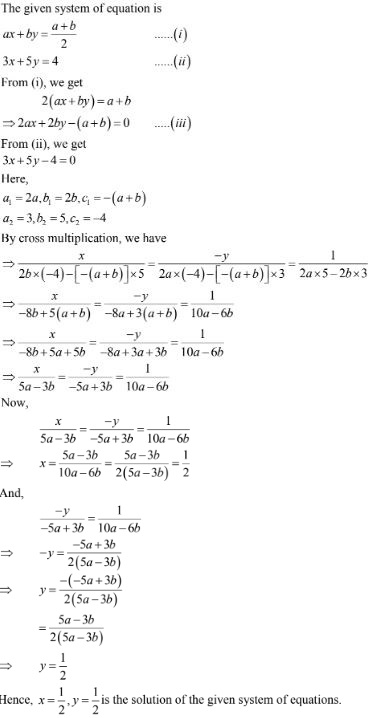15. 2ax + 3by = a + 2b
3ax + 2by = 2a + b

Solution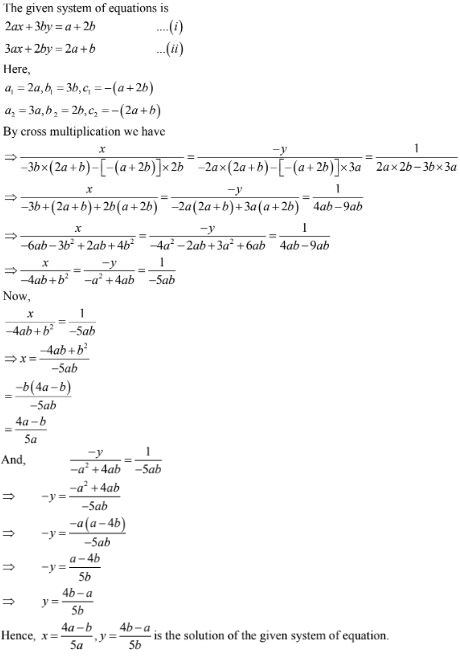16. 5ax + 6by = 28
3ax + 4by =
18

Solution17. (a + 2b)x + (2a - b)y = 2
(a - 2b)x + (2a + b)y = 3

Solution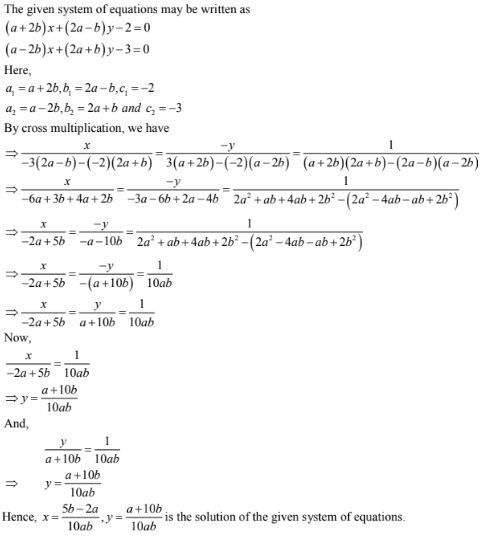18. x(a- b + ab/a-b) = y(a+ b - ab/a+b)
x + y = 2a2

Solution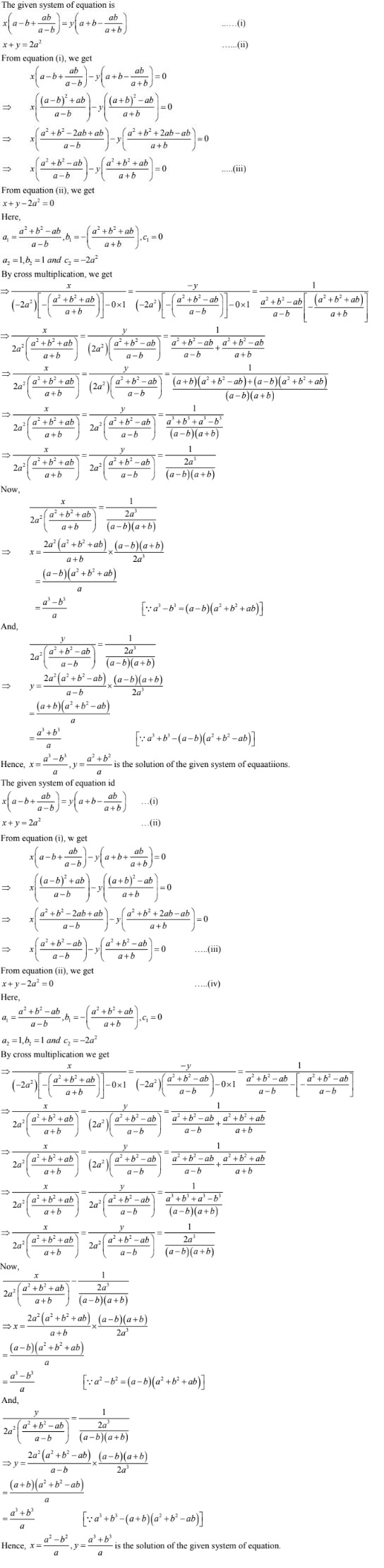19. ax[1/(a-b) - 1/(a+b)] + cy[1/(b-a) - 1/(b+a)] = 2a/(a+b)

Solution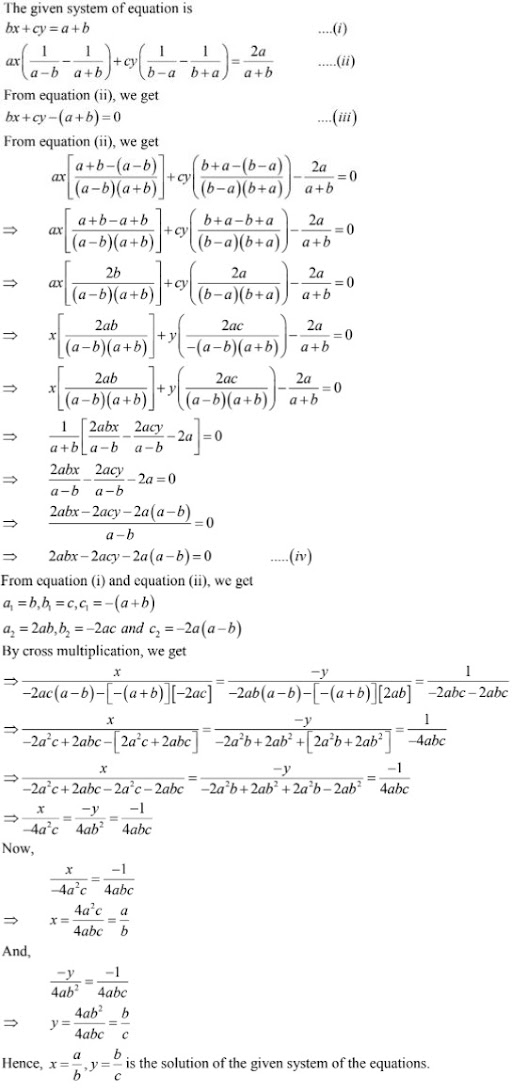20. (a - b)x + (a+ b)y = 2a2  -  2b2
(a+b)(x+y) = 4ab

Solution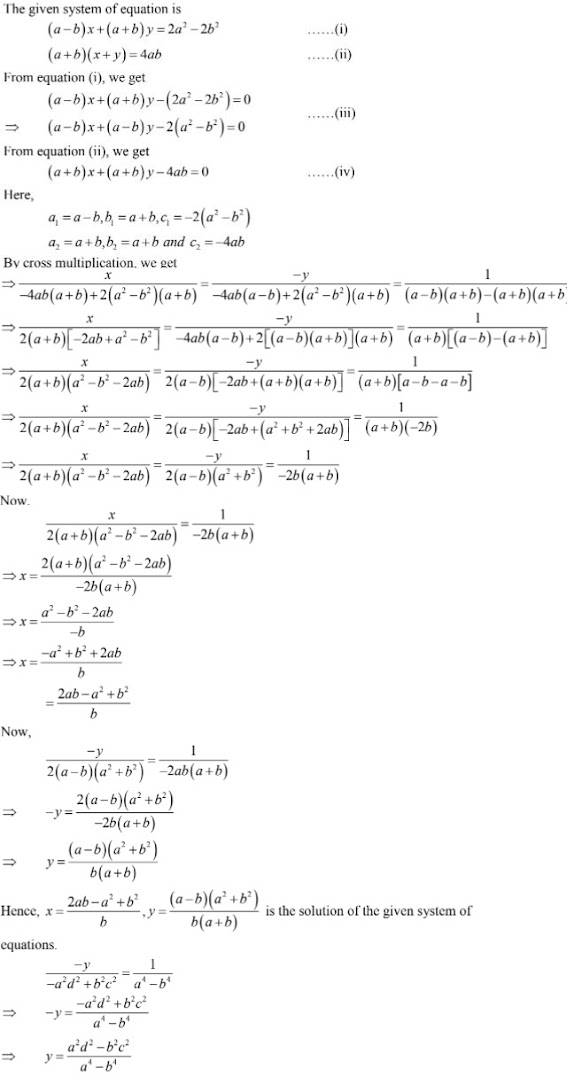21. a2x + b2y = c2
b2x + a2y = d2

Solution

The given system of equations may be written as
a2x + b2y - c2  = 0
b2x + a2y - d2  = 0
Here,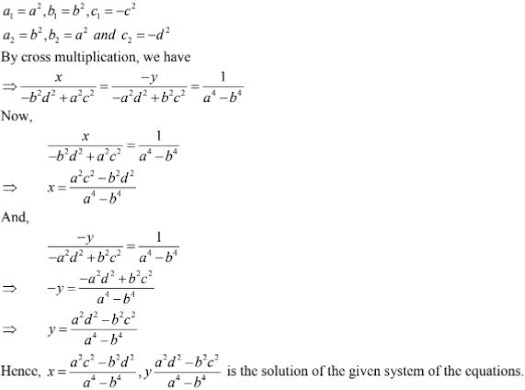22. 57/(x+y) + 6/(x-y) = 5
38/(x+y) + 21/(x-y) = 9

Solution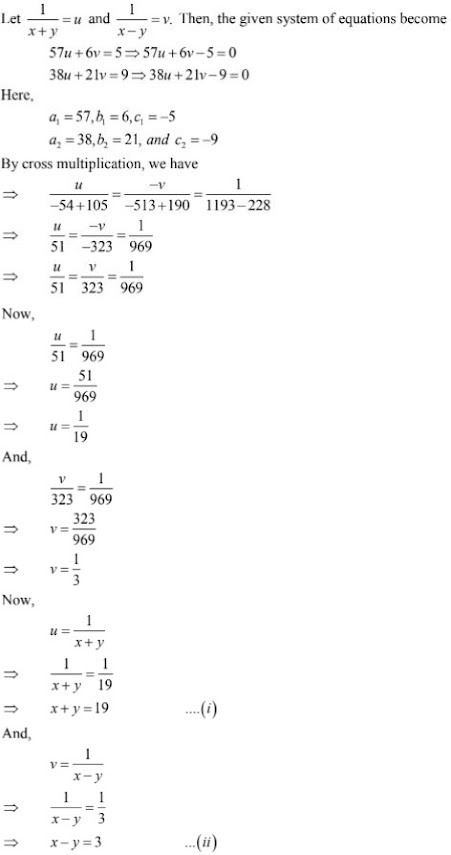23. 2(ax - by) + a + 4b = 0
2(bx + ay) + b - 4a = 0

Solution

The given system of equation may be written as
2ax - 2by + a +4b = 0
2bx + 2ay + b - 4a = 0
Here,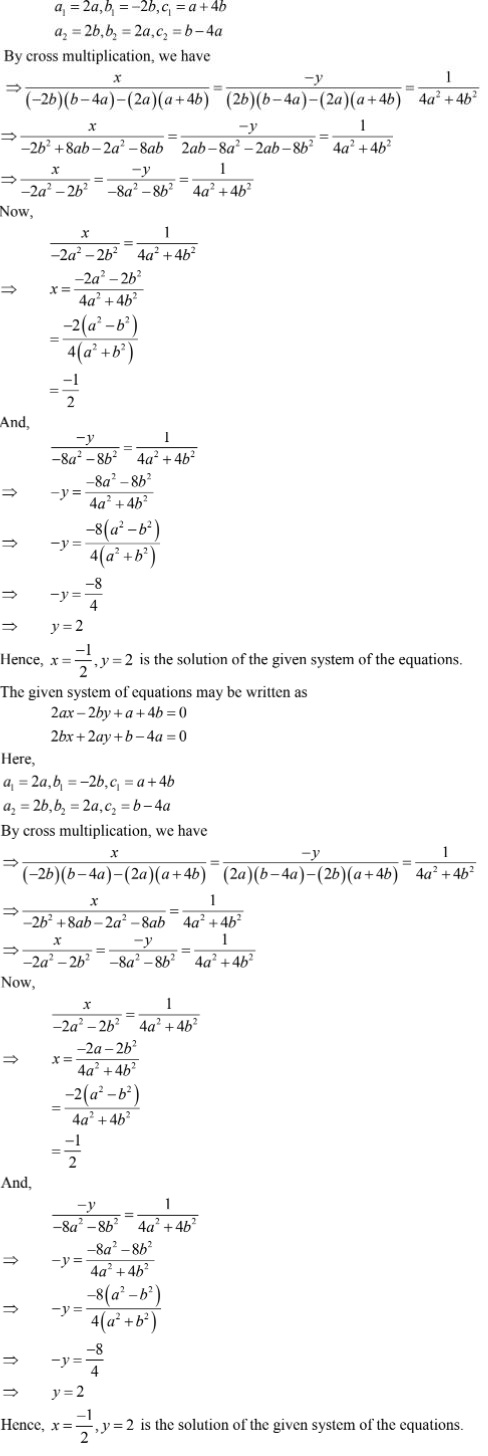24. 6(ax + by) = 3a + 2b
6(bx - ay) = 3b - 2a

Solution

The given system of equation is
6(ax + by) = 3a + 2b ...(i)
6(bx - ay) = 3b - 2a ...(ii)
From equation (i), we get
6ax + 6by - (3a + 2b) = 0 ...(iii)
From equation (ii), we get
6bx - 6ay - (3b - 2a) = 0 ...(iv)
Here,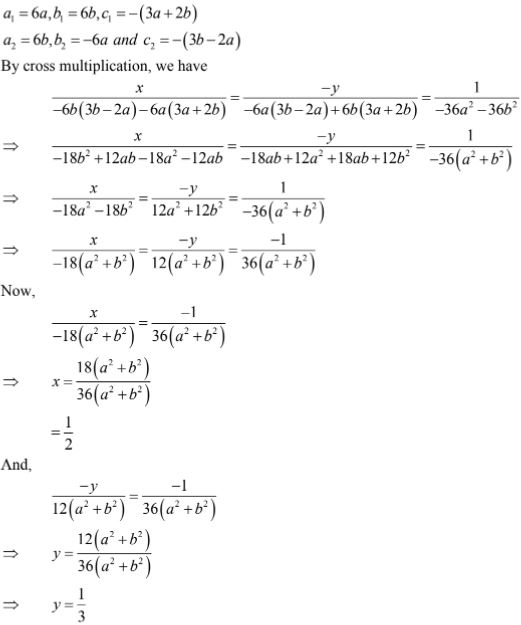Hence, x = 1/2, y = 1/3  is the solution of the given system of equations.

25. a2/x - b2/y = 0
a2b/x + b2a/y = a + b, x, y ≠ 0

Solution

Taking 1/x = u and 1/y = v. Then, the given system of equations become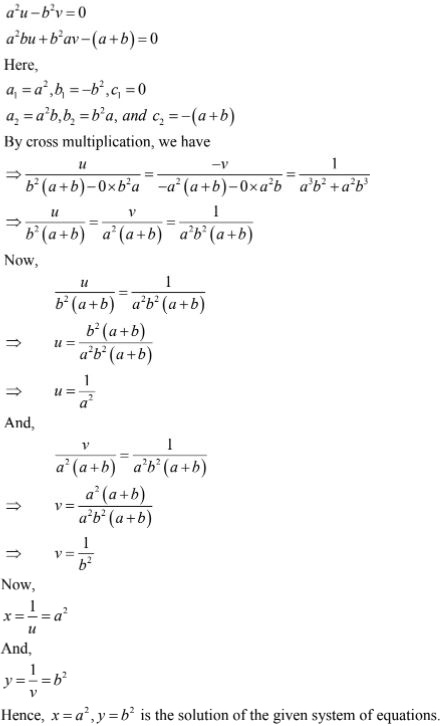26. mx - my = m2  + n2
x + y = 2m

Solution

The given system of equations may be written as
mx - ny - (m2  + n2) = 0
x + y - 2m = 0
Here,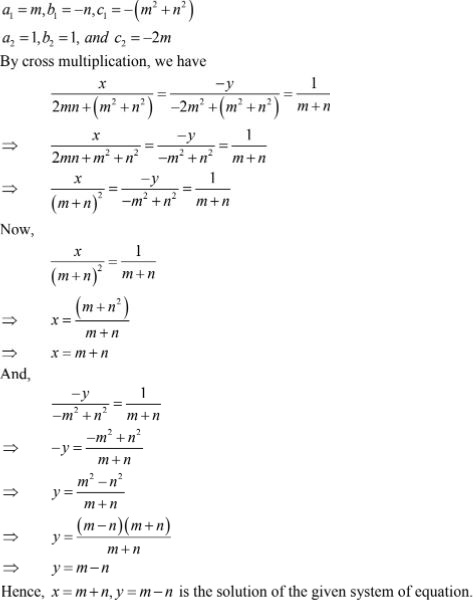27. ax/b - by/a = a+ b
ax - by = 2ab

Solution

The given system of equation may be written as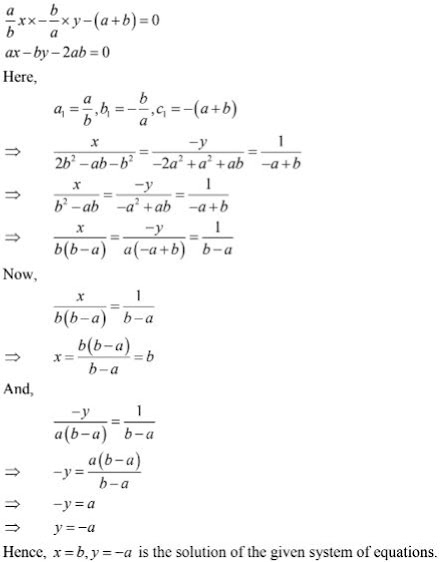28.(b/a)x + (a/b)y - (a2  + b2) = 0
x + y - 2ab = 0

Solution